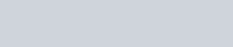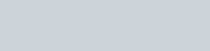# Linear Regression with Automatic Differentiation using TensorFlow in Python

Welcome all, in this article, we will work on Linear Regression with Automatic Differentiation using TensorFlow. In this course, we will be focusing on the following learning objectives:

• Tensor constant and variables in Tensorflow
• Automatic differentiation in Tensorflow
• Solving linear regression problem using automatic differentiation in Tensorflow

Before starting you must install all the required libraries mentioned below. And for the best working experience, Jupyter notebook is recommended. (To install it you can visit this link- https://jupyter.org/install )

## Importing The Python Libraries

We will import all the necessary libraries.

```import tensorflow as tf
import numpy as np
import matplotlib.pyplot as plt
%matplotlib inline```

## Tensor Constants

We will use two functions ( i.e. constant() and convert_to_tensor()) to create the tensor constants.

In the below code, we are passing a list with values = 10,20,30 to the constant() function in Tensorflow.

```tf.constant([[10,20,30]])
```

Output:

`<tf.Tensor: id=0, shape=(1, 3), dtype=int32, numpy=array([[10, 20, 30]])>`

Here, you can find the shape, data type, and values associated with the constant.

In the below code, we are passing a list with values = 10,20,30 to the convert_to_tensor() function in Tensorflow.

`tf.convert_to_tensor([[10, 20, 30]])`

Output:

`<tf.Tensor: id=1, shape=(1, 3), dtype=int32, numpy=array([[10, 20, 30]])>`

You can see it is similar to the above output but here, we have used convert_to_tensor() function.

`tf.convert_to_tensor([[10, 20, 30]], dtype=tf.float32)`

Output:

`<tf.Tensor: id=2, shape=(1, 3), dtype=float32, numpy=array([[10., 20., 30.]], dtype=float32)>`

In this code, we changed the data type to float32 from int32.

`tf.convert_to_tensor([[10, 20, 30]]).numpy()`

Output:

`array([[10, 20, 30]])`

Using numpy() function we have passed the values to create a NumPy array.

## Tensor Variables

Here we will learn to create tensor variables and also perform matrix multiplication with the tensor constants and variables.

In the below code, we are passing a list with values = 10,20,30 to the Variable() function in Tensorflow which will create a variable NumPy array.

`tf.Variable([[10, 20, 30]])`

Output:

`<tf.Variable 'Variable:0' shape=(1, 3) dtype=int32, numpy=array([[10, 20, 30]])>`

Now, let us see the use of Variable() function and how can we assign different values to the same variable.

```v = tf.Variable(5)
print('Initial value:', v.numpy())
v.assign(10)
print('New value:', v.numpy())```

Output:

```Initial value: 5
New value: 10```

In the above code, variable v is assigned a value 5 initially, and in the next line its changed t0 10 using the assign() function.

So now, Let’s do the Matrix Multiplication :

```c = tf.convert_to_tensor(np.random.randn(2, 3))
v = tf.Variable(np.random.randn(3, 1))

print(tf.matmul(c, v))```

In the above code we have taken a tensor constant and a tensor variable of dimensions (2, 3) and (3, 1) respectively. So to take the input values we have used random() function which will pass any random value to the variables. And after that, we have used matmul() function in Tensorflow to calculate the matrix multiplication of c and v.

Output:

```tf.Tensor(
[[1.27194381]
[0.86044265]], shape=(2, 1), dtype=float64)```

This is the solution to the matrix multiplication. Don’t worry if you get a different output, since we are using random() function, we will get a different value for every execution.

## Automatic Differentiation

Let’s take a simple equation:So in the below code we have taken a variable x and assigned a value to it which is 3 here. Next, using GradientTape() we are performing automatic differentiation in Tensorflow. We have assigned y=x**3 (which is our base equation). Next, using tape.gradient we got our first differentiation. (Note: Here we have assigned 3 but you can check the answer for different values of x.)

```x = tf.Variable(3.0)

y = x**3

print('gradient at x={} is {}'.format(x.numpy(), dy_dx.numpy()))```

Output:

`gradient at x=3.0 is 27.0`

Again, Let us see higher-order gradients:Similarly to the first-order differentiation, here also we will use GradientTape(). So for second-order differentiation, we have defined two GradientTape() as t1 and t2. Under this, we have calculated the first and second-order derivatives.

```x = tf.Variable(3.0)

y = x**3

print('2nd order gradient at x={} is {}'.format(x.numpy(), d2y_dx2.numpy()))```

Output:

`2nd order gradient at x=3.0 is 18.0`

## More Examples of Automatic Differentiation

We all know when we try to differentiate a constant we get nothing. so below example shows exactly what happens when a constant is used in GradientTape().

```x = tf.constant(3.0)

y = x**3

print(dy_dx)```

Output:

`None`

So to resolve the above problem we have to use watch() function. This is will treat the constant as a variable in Tensorflow and will calculate the equation under it. You all can see the output which will show the correct answer and not None this time.

```x = tf.constant(3.0)

tape.watch(x)
y = x**3

print(dy_dx)```

Output:

`tf.Tensor(27.0, shape=(), dtype=float32)`

## Persistent TapeWe can call tape.gradient() only once, So to use this multiple times we have to pass “persistent=True” as the parameter. But with this, we have to delete the tape after using it since it could be dangerous sometimes.

```x = tf.Variable(3.0)

y = x**3
z = 2*y

del tape

print('dz_dy =', dz_dy.numpy())
print('dy_dx =', dy_dx.numpy())
print('dz_dx =', dz_dx.numpy())

print('dz_dx =', dy_dx.numpy() * dz_dy.numpy())```

Output:

```dz_dy = 2.0
dy_dx = 27.0
dz_dx = 54.0
dz_dx = 54.0```

let’s now move to solve a simple linear equation using automatic differentiation.

## Generating Data for Linear Regression

So now, Let’s take a simple linear regression equation:Here, we are creating a batch of 64 values using true values of w and b as 7 and 4 respectively. The function create_batch() will generate the required values. And to add some randomness to our solution we are using random() function.

```true_w, true_b = 7.0, 4.0

def create_batch(batch_size=64):
x = np.random.randn(batch_size, 1)
y = np.random.randn(batch_size, 1) + true_w * x + true_b

return x, y```
```x, y = create_batch()

plt.plot(x, y, '.');```

Output:In the graph, you can see it is depicting a straight line with some random values which shows that it is a linear equation.

## Linear Regression

In the final phase, we just have to implement all those things which we have already learned earlier.

We have taken number of iterations as 100 and the learning rate(lr) is set to 0.03. After declaring the tensor constant w and b we are next finding the value of y under the GradientTape(). Then we are focusing to calculate the mean squared error using the square() and reduced_mean() method. Now using automatic differentiation we will assign the new values to w and b and after 10 iterations we are printing them. Thus you can see how our model is learning.

```iterations = 100
lr = 0.03

w = tf.Variable(10.0)
b = tf.Variable(1.0)

param_history = {'w': [], 'b': []}

for i in range(0, iterations):
x_batch, y_batch = create_batch()
x_batch = tf.constant(x_batch, dtype=tf.float32)
y_batch = tf.constant(y_batch, dtype=tf.float32)
y = b + w * x_batch
loss = tf.reduce_mean(tf.square(y - y_batch))

del tape

w.assign_sub(lr * dw)
b.assign_sub(lr * db)

param_history['w'].append(w.numpy())
param_history['b'].append(b.numpy())

if i%10==0:
print('At iter {}, w={}, b={}'.format(i, w.numpy(), b.numpy()))```

Output:

```At iter 0, w=9.871705055236816, b=1.1615238189697266
At iter 10, w=8.51032543182373, b=2.4824905395507812
At iter 20, w=7.811744213104248, b=3.1616461277008057
At iter 30, w=7.431243896484375, b=3.577282428741455
At iter 40, w=7.2762956619262695, b=3.7577056884765625
At iter 50, w=7.16446590423584, b=3.87068772315979
At iter 60, w=7.0791168212890625, b=3.9401323795318604
At iter 70, w=7.022558212280273, b=3.9625468254089355
At iter 80, w=7.063626766204834, b=3.9933183193206787
At iter 90, w=7.03794002532959, b=3.9649362564086914```

In the below graph you can see how the values of w and b are changing to true values(7 and 4) as the number of iterations is increasing.

```plt.figure(figsize=(6, 6))
plt.plot(range(iterations), param_history['w'], label='Learned W')
plt.plot(range(iterations), param_history['b'], label='Learned b')
plt.plot(range(iterations), [true_w]*iterations, label='True W')
plt.plot(range(iterations), [true_b]*iterations, label='True b')
plt.xlabel('Training Iterations')
plt.ylabel('Value')
plt.legend()
plt.show()```

Output plot will be:Initially, we have assigned w=10 and b=1. So here you can see that after 100 iterations the values are almost changed to true values i.e. w=7 and b=4.

Thank you!!

### One response to “Linear Regression with Automatic Differentiation using TensorFlow in Python”

1.Aditi Shukla says:

Article was well written and beautifully explained.